[Introduction]  [Signal arithmetic]  [Signals and noise]   [Smoothing]   [Differentiation]  [Peak Sharpening]  [Harmonic analysis]   [Fourier convolution]  [Fourier deconvolution]  [Fourier filter]  [Wavelets]   [Peak area measurement]  [Linear Least Squares]  [Multicomponent Spectroscopy]  [Iterative Curve Fitting]  [Hyperlinear quantitative absorption spectrophotometry] [Appendix and Case Studies]  [Peak Finding and Measurement]  [iPeak]   [iSignal]  [Peak Fitters]   [iFilter]  [iPower]  [List of downloadable software]  [Interactive tools]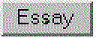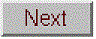Combining Methods: Hyperlinear Quantitative Absorption Spectrophotometry

[Background]  [Spreadsheets]  [Matlab/Octave functions] [Simple example] [Simple TFit Demo]  [Demonstration scripts]  [Download ZIP file]  [Have a question? Email me]  [Interactive tools]

This example shows how knowledge of the specific optical measurement system can be used to design a custom signal processing procedure, constructed by combining several of the methods and concepts introduced in this essay, that expands the classical limits of measurement in optical spectroscopy. This is a computational method for quantitative analysis by multiwavelength absorption spectroscopy, called the transmission-fitting or "TFit" method, which is based on fitting a model of the instrumentally-broadened transmission spectrum to the observed transmission data, rather than direct "classical" calculation of absorbance as log10(Io/I). The method is described in: T. C. O'Haver and J. Kindervater, J. of Analytical Atomic Spectroscopy 1, 89 (1986); T. C. O'Haver and Jing-Chyi Chang, Spectrochim. Acta 44B, 795-809 (1989); T. C. O'Haver, Anal. Chem. 68, 164-169 (1991). It is included as an example of combining techniques because it uses several important concepts that have been covered in this essay: signal-to-noise ratio, Fourier convolution, multcomponent spectroscopy, iterative least-squares fitting, and calibration.

Advantages of the TFit method compared to conventional methods are:

(a) much wider dynamic range (i.e., the concentration range over which one calibration curve can be expected to give good results) ,

(b) greatly improved calibration linearity ("hyperlinearily"), which reduces the labor and cost of preparing and running large numbers of standard solutions and safely disposing of them afterwards, and

(c) the ability to operate under conditions that are optimized for signal-to-noise ratio rather than for Beer's Law ideality, that is, small spectrometers with shorter focal length, lower dispersion and larger slit widths to increase light throughput and reduce the effect of photon and detector noise (assuming, of course, that the detector is not saturated or overloaded).

Just like the multilinear regression (classical least squares) methods conventionally used in absorption spectroscopy, the Tfit method

(a) requires an accurate reference spectrum of each analyte,

(b) utilizes multiwavelength data such as would be acquired on diode-array, Fourier transform, or automated scanning spectrometers,

(c) applies both to single-component and multicomponent mixture analysis, and

(d) requires that the absorbances of the components vary with wavelength, and, for multi-component analysis, that the absorption spectra of the components be sufficiently different. Black or grey absorbers do not work with this method.

The disadvantages of the Tfit method are:

(a) it makes the computer work harder than the multilinear regression methods (but, on a typical personal computer, calculations take only a fraction of a second, even for the analysis of a mixture of several components);

(b) it requires knowledge of the instrument function, i.e., the slit function or the resolution function of an optical spectrometer (however, this is a fixed characteristic of the instrument and can be measured beforehand by scanning the spectrum of a narrow atomic line source such as a hollow cathode lamp). It changes only if you change the slit width of the spectrometer;

(c) it is an iterative method that can under unfavorable circumstances converge on an incorrect local optimum, but this is handled by proper selection of the starting values calculated by the traditional log (Io/I) method), and

(d) It won't work for gray-colored substances whose absorption spectra do not vary over the spectral region measured.

The following sections give the background of the method and a description of the main function and demonstration programs and spreadsheet templates:

Background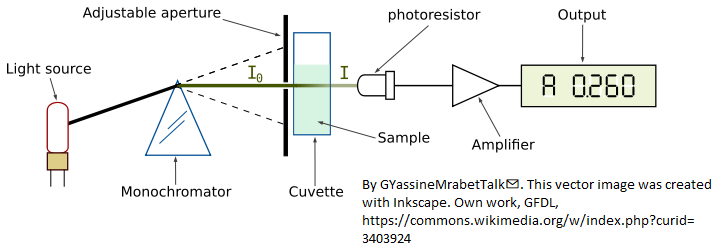In optical absorption spectroscopy, the intensity I of light passing through an absorbing sample is given (in Matlab notation) by the Beer-Lambert Law:
I = Io.*10^-(alpha*L*c)

where "Io" (pronounced "eye-zero") is the intensity of the light incident on the sample, "alpha" is the absorption coefficient of the absorber, "L" is the distance that the light travels through the material (the path length), and "c"' is the concentration of absorber in the sample. The variables I, Io, and alpha are all functions of wavelength; L and c are scalar.

In conventional applications, measured values of I and Io are used to compute the quantity called "absorbance", defined as

A = log10(Io/I)

Absorbance is defined in this way so that, when you combine this definition with the Beer-Lambert law, you get:

A = alpha*L*c

So, absorbance is proportional to concentration, ideally, which simplifies analytical calibration. However, any real spectrometer has a finite spectral resolution, meaning that the light beam passing through the sample is not truly monochromatic, with the result that an intensity reading at one wavelength setting is actually an average over a small spectral interval. More exactly, what is actually measured is a convolution of the true spectrum of the absorber and the instrument function, the instrument's response as a function of wavelength of the light. Ideally the instrument function is infinitely narrow (a "delta" function), but practical spectrometers have a non-zero slit width, the width of the adjustable aperture in the diagram above, which passes a small spectral interval of wavelengths of light from the dispersing element (prism) onto the sample and detector. If the absorption coefficient "alpha" varies over that spectral interval, then the calculated absorbance will no longer be linearly proportional to concentration (this is called the "polychromicity" error). The effect is most noticeable at high absorbances. In practice, many instruments will become non-linear starting at an absorbance of 2 (~1% Transmission). As the absorbance increases, the effect of unabsorbed stray light and instrument noise also becomes more significant.

The theoretical best signal-to-noise ratio and absorbance precision for a photon-noise limited optical absorption instrument can be shown to be close to an absorbance close to 1.0 (see Is there an optimum absorbance for best signal-to-noise ratio?). The range of absorbances below 1.0 is easily accessible down to at least 0.001, but the range above 1.0 is limited. Even an absorbance of 10 is unreachable on most instruments and the direct measurement of an absorbance of 100 is unthinkable, as it implies the measurement of light attenuation of 100 powers of ten - no real measurement system has a dynamic range even close to that. In practice, it is difficult to achieve an dynamic range even as high as 5 or 6 absorbance, so that much of the theoretically optimum absorbance range is actually unusable. (c.f. http://en.wikipedia.org/wiki/Absorbance). So, in conventional practice, greater sample dilutions and shorter path lengths are required to force the absorbance range to lower values, even if this means poorer signal-to-noise ratio and measurement precision at the low end.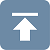It is true that the non-linearity caused by polychromicity can be reduced by operating the instrument at the highest resolution setting (reducing the instrumental slit width). However, this has a serious undesired side effect: in dispersive instruments, reducing the slit width to increase the spectral resolution degrades the signal-to-noise substantially. It also reduces the number of atoms or molecules that are actually measured. Here's why: UV/visible absorption spectroscopy is based on the the absorption of photons of light by molecules or atoms resulting from transitions between electronic energy states. It's well known that the absorption peaks of molecules are more-or-less wide bands, not monochromatic lines, because the molecules are undergoing vibrational and rotational transitions as well and are also under the perturbing influence of their environment. This is the case also in atomic absorption spectroscopy: the absorption "lines" of gas-phase free atoms, although much narrower that molecular bands, have a finite non-zero width, mainly due to their velocity (temperature or Doppler broadening) and collisions with the matrix gas (pressure broadening). A macroscopic collection of molecules or atoms, therefore, presents to the incident light beam a distribution of energy states and absorption wavelengths. Absorption results from the collective interaction of many individual atoms or molecules with individual photons. A purely monochromatic incident light beam would have photons all of the same energy, ideally corresponding to the average energy distribution of the collection of atoms or molecules being measured. But many - actually most - of the atoms or molecules would have a energy greater or less than the average and would thus not be measured.  If the bandwidth of the incident beam is increased, more of those non-average atoms or molecules would be available to be measured, but then the simple calculation of absorbance as log10(Io/I) is no longer valid and would result in a non-linear response to concentration.

Numerical simulations show that the optimum signal-to-noise ratio is typically achieved when the spectral resolution of the instrument approximately matches the width of the analyte absorption, but then using the conventional log10(Io/I) absorbance would result in very substantial non-linearity over most of the absorbance range because of the polychromicity error. This non-linearity has its origin in the spectral domain (intensity vs wavelength), not in the calibration domain (absorbance vs concentration). Therefore it should be no surprise that curve fitting in the calibration domain, for example fitting the calibration data with a quadratic or cubic fit, would not be the best solution, because there is no theory that says that the deviations from linearity would be expected to be exactly quadratic or cubic. A more theory-based approach would be to perform the curve fitting in the spectral domain where the source of the non-linearity arises.This is possible with modern absorption spectrophotometers that use array detectors, which have many tiny detector elements that slice up the spectrum of the transmitted beam into many small wavelength segments, rather than detecting the sum of all those segments with one big photo-tube detector, as older instruments do. An instrument with an array detector typically uses a slightly different optical arrangement, as shown by the simplified diagram on the left, where the spectral resolution is determined by both the entrance slit and the range of optical detector elements that are summed to determine the transmitted intensity.

The TFit method sidesteps the above problems by calculating the absorbance in a completely different way: it starts with the reference spectra (an accurate absorption spectrum for each analyte, also required by the multilinear regression methods), normalizes them to unit height, multiplies each by an adjustable coefficient - initially equal to the conventional log10(Io/I) absorbance - adds them up, computes the antilog, and convolutes it with the previously-measured slit function. The result, representing the instrumentally broadened transmission spectrum, is compared to the observed transmission spectrum. The coefficients (one for each unknown component in the mixture) are adjusted by the program until the computed transmission model is a least-squares best fit to the observed transmission spectrum. The best-fit coefficients are then equal to the absorbances determined under ideal optical conditions. Provision is also made to compensate for unabsorbed stray light and changes in background intensity (background absorption). These calculations are performed by the function fitM, which is used as a fitting function for Matlab's iterative non-linear fitting function fminsearch. The TFit method gives measurements of absorbance that are much closer to the "true" peak absorbance that would have been measured in the absence of stray light and polychromatic light errors. More important, it allows linear and wide dynamic range measurements to be made even if the slit width of the instrument is increased to optimize the signal-to-noise ratio.

From a historical perspective, by the time Pierre Bouguer discovered what became to be known as the Beer-Lambert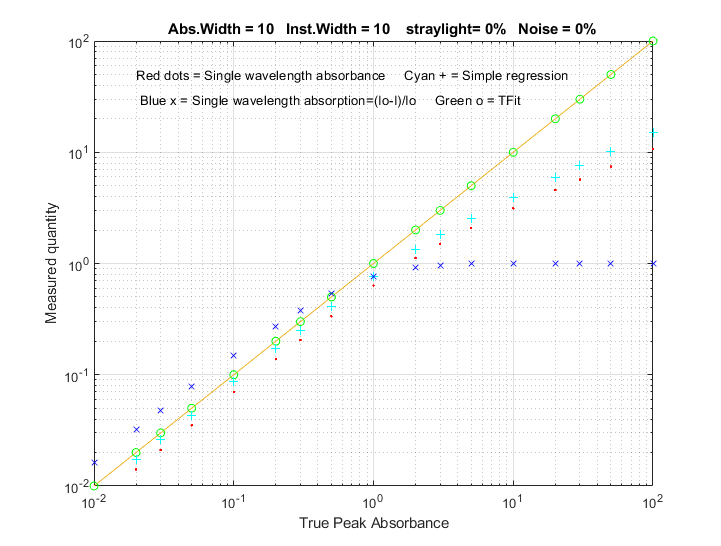law in 1729, the logarithm was already well known, having been introduced by John Napier in 1614. So the additional mathematical work needed to compute the absorbance, log(Io/I), rather than the simpler relative absorption, (Io-I)/Io, was justified because of the better linearity of absorbance with respect to concentration and path length, and the calculation could easily be performed simply by a slide-rule type graduated scale. Certainly by today's standards, the calculation of a logarithm is considered routine. In contrast, the TFit method presented here is far more mathematically complex than a logarithm and cannot be done without the aid of software (at least a spreadsheet) and some sort of computational hardware, but it offers a further improvement in linearity beyond that achieved by the logarithmic calculation of absorbance, and it additionally allows the small slit width limitation to be loosened. The figure on the right compares the analytical curve linearity of simple relative absorption (blue x), logarithmic absorbance (red dots), multilinear regression or CLS method (cyan +) based on absorbance, and the TFit method (green o). This plot was created by the Matlab/Octave script TFitCalCurveAbs.m.

Bottom line: The TFit method is based on the Beer-Lambert Law; it simply calculates the absorbance in a different way that does not require the assumption that stray light and polychromatic radiation effects are zero. Because it allows larger slit widths and shorter focal lengths to be used, it yields greater signal-to-noise ratios while still achieving a much wider linear dynamic range than previous methods, thus requiring fewer standards to properly define the calibration curve and avoiding the need for non-linear calibration models. Keep in mind that the log(Io/I) absorbance calculation is a 165-year-old simplification that was driven by the need for mathematical convenience, and by the mathematical skills of the college students to whom this subject is typically first presented, not by the desire to optimize detection sensitivity and signal-to-noise ratio. It dates from the time before electronics and computers, when the only computational tools were pen and paper and slide rules, and when a method such as described here would have been unthinkable. That was then; this is now. Tfit is the 21st century way to do quantitative absorption spectrophotometry.

Note: The TFit method compensates for the non-linearity caused by unabsorbed stray light and the polychromatic light effect, but other potential sources of non-linearity remain, in particular chemical effects, such as photolysis, equilibrium shifts, temperature and pH effects, binding, dimerization, polymerization, molecular phototropism, fluorescence, etc. A well-designed quantitative analytical method is designed to minimize those effects.

The Tfit method can also be implemented in an Excel or Calc spreadsheet; it's a bit more cumbersome that the Matlab/Octave implementation, but it works. The shift-and-multiply method is used for the convolution of the reference spectrum with the slit function, and the "Solver" add-in for Excel and Calc is used for the iterative fitting of the model to the observed transmission spectrum. This external source, https://www.wallstreetmojo.com/solver-in-excel/, has a detailed graphical explanation of using the Solver. It's very handy, but not essential, to have a "macro" capability to automate the process (See http://peltiertech.com/Excel/SolverVBA.html#Solver2 for more info about setting up macros and solver on your version of Excel).

TransmissionFittingTemplate.xls (screen image) is an empty template; all you have to do is to enter the data in the cells marked by a gray background: wavelength (Column A), observed absorbance of the sample (Column B), the high-resolution reference absorbance spectrum (Column D), the stray light (A6) and the slit function used for the observed absorbance of the sample (M6-AC6). (TransmissionFittingTemplateExample.xls (screen image) is the same template with example data entered.
TransmissionFittingDemoGaussian.xls (screen image) is a demonstration with a simulated Gaussian
absorption peak with variable peak position, width, and height, plus added stray light, photon noise, and detector noise, as viewed by a spectrometer with a triangular slit function. You can vary all the parameters and compare the best-fit absorbance to the true peak height and to the conventional log(1/T) absorbance.
All of these spreadsheets include a macro, activated by pressing Ctrl-f, that uses the Solver function to perform the iterative least-squares calculation (see CaseStudies.html#Using_Macros). But if you prefer not to use macros, you can do it manually by clicking the Data tab, Solver, Solve, and then OK.

TransmissionFittingCalibrationCurve.xls (screen image) is a demonstration spreadsheet that includes another Excel macro that constructs calibration curves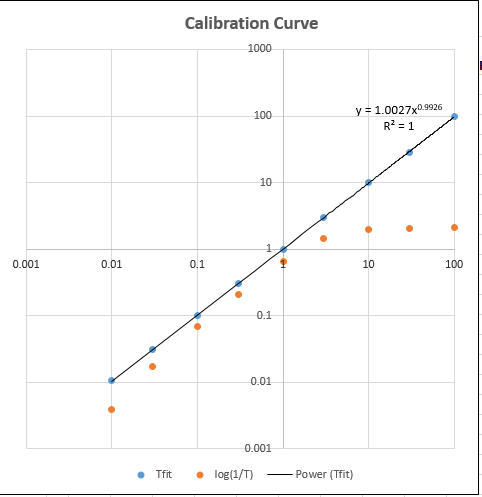comparing the TFit and conventional log(1/T) methods for a series of 9 standard concentrations that you can specify. To create a calibration curve, enter the standard concentrations in AF10 - AF18 (or just use the ones already there, which cover a 10,000-fold concentration range from 0.01 to 100), then press Ctrl-f to run the macro. In this spreadsheet the macro does a lot more than in the previous example: it automatically goes through the first row of the little table in AF10 - AH18, extracts each concentration value in turn, places it in the concentration cell A6, recalculates the spreadsheet, takes the resulting conventional absorbance (cell J6) and places it as the first guess in cell I6, brings up the Solver to compute the best-fit absorbance for that peak height, places both the conventional absorbance and the best-fit absorbance in the table in AF10 - AH18, then goes to the next concentration and repeats for each concentration value. Then it constructs and plots the log-log calibration curve (shown on the right) for both the TFit method (blue dots) and the conventional (red dots) and computes the trend-line equation and the R2 value for the TFit method, in the upper right corner of graph. Each time you press Ctrl-f it repeats the whole calibration curve with another set of random noise samples. (Note: you can also use this spreadsheet to compare the precision and reproducibility of the two methods by entering the same concentration 9 times in AF10 - AF18. The result should ideally be a straight flat line with zero slope).

Matlab/Octave implementation: The fitM.m functionfunction err = fitM(lam,yobsd,Spectra,InstFun,StrayLight)

fitM is a fitting function for the Tfit method, for use with Matlab's or Octave's fminsearch function. The input arguments of fitM are:

absorbance= vector of absorbances that are calculated to give the best fit to the transmission spectrum.
yobsd = observed transmission spectrum of the mixture sample over the spectral range (column vector)
Spectra = reference spectra for each component, over the same spectral range, one column/component, normalized to 1.00.
InstFun = Zero-centered instrument function or slit function (column vector)
StrayLight = fractional stray light (scalar or column vector, if it varies with wavelength)
Note: yobsd, Spectra, and InstFun must have the same number of rows (wavelengths). Spectra must have one column for each absorbing component.

Typical use:

absorbance = fminsearch(@(lambda)(fitM(lambda, yobsd, TrueSpectrum, InstFun, straylight)), start);

where start is the first guess (or guesses) of the absorbance(s) of the analyte(s); it's convenient to use the conventional log10(Io/I) estimate of absorbance for start. The other arguments (described above) are passed on to FitM. In this example, fminsearch returns the value of absorbance that would have been measured in the absence of stray light and polychromatic light errors (which is either a single value or a vector of absorbances, if it is a multi-component analysis). The absorbance can then be converted into concentration by any of the usual calibration procedures (Beer's Law, external standards, standard addition, etc.).Here is a specific numerical example, for a single-component measurement where the true absorbance is 1.00, using only 4-point spectra for illustrative simplicity (of course, array-detector systems would acquire many more wavelengths than that, but the principle is the same). In this particular case the instrument width (InstFun) is twice the absorption width, the stray light is constant at 0.01 (1%). The conventional single-wavelength estimate of absorbance is too low: log10(1/.38696)=0.4123. In contrast, the TFit method using fitM:

yobsd=[0.56529 0.38696 0.56529 0.73496]';
TrueSpectrum=[0.2 1 0.2 0.058824]';
InstFun=[1 0.5 0.0625 0.5]';
straylight=.01;
start=.4;
absorbance=fminsearch(@(lambda)(fitM(lambda,
yobsd,TrueSpectrum,InstFun,straylight)),start)

returns the correct value of 1.000. (The "start" value, which is the conventional log10(Io/I) absorbance, is not critical and can be just about any value you like). For a multiple-component measurement, the only difference is that the variable "TrueSpectrum" would be a matrix rather than a vector, with one column for each absorbing component. The resulting "absorbance" would then be a vector rather than a single number, with one absorbance value for each component. (See TFit3.m below for an example of a 3-component mixture).

Iterative least-squares methods are ordinarily considered to be more difficult and less reliable than multilinear regression methods, and this can be true if there are more than one nonlinear variable that must be iterated, especially if those variables are correlated.  However, in the TFit method, there is only one iterated variable (absorbance) per measured component, and reasonable first guesses are readily available from the conventional single-wavelength absorbance calculation or multiwavelength regression methods. As a result, an iterative least-squares method works very well in this case. The expression for absorbance given above for the TFit method can be compared to that for the weighted regression method:

absorbance=([weight weight].*[Background RefSpec])\(-log10(yobsd).*weight)
where RefSpec is the matrix of reference spectra of all of the pure components. You can see that, in addition to the RefSpec and observed transmission spectrum (yobsd), the TFit method also requires a measurement of the Instrument function (spectral bandpass) and the stray light (which the linear regression methods assume to be zero), but these are characteristics of the spectrometer and need be done only once for a given spectrometer.

Finally, although the TFit method does make the computer work harder, the computation time on a typical laboratory personal computer is only a fraction of a second (roughly 25 microsec per spectral data point per component analyzed), using Matlab as the computational environment. The cost of the computational hardware need not be burdensome; the method can even be performed on a \$35 single-board computer.

The tfit.m demonstration function for Matlab or Octave.The tfit.m function is a self-contained command-line demonstration function that compares the TFit method to the single-wavelength (SingleW), simple regression (SimpleR), and weighted regression (WeightR) methods. The syntax is tfit(absorbance), where 'absorbance' is the true peak absorbance (True A) of an absorber with a Lorentzian spectral profile of width 'width' (line 29), measured with a spectrometer with a Gaussian spectral bandpass of width 'InstWidth' (line 30), fractional unabsorbed stray light level of 'straylight' (line 32), photon noise level of 'noise' (line 31) and a random Io shift of 'IoShift' (line 33).  Plots the spectral profiles and prints the measured absorbances of each method in the command window.  Examples:

>> tfit(1)

width = 10
InstWidth = 20
noise = 0.01
straylight = 0.01
IzeroShift = 0.01

True A    SingleW    SimpleR     WeightR    TFit
1      0.38292     0.54536     0.86839    1.0002

>> tfit(10)
10     1.4858      2.2244      9.5123     9.9979

>> tfit(100)
100     2.0011      3.6962      57.123     99.951

>> tfit(200)
200     2.0049      3.7836      56.137    200.01

>> tfit(.001)
0.001   0.00327     0.00633     0.000520    0.000976

TFitDemo.m: Interactive explorer for the Tfit method, version 2.1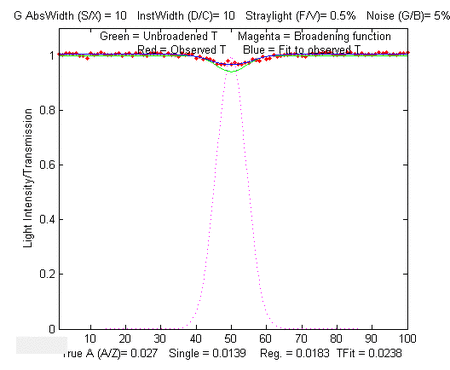Keypress-operated interactive explorer for the Tfit method, applied to the measurement of a single component with a Lorentzian (or Gaussian) absorption peak, with controls that allow you to adjust the true absorbance (Peak A), spectral width of the absorption peak (AbsWidth), spectral width of the instrument function (InstWidth), stray light, and the photon noise level (Noise) continuously while observing the effects graphically and numerically. Simulates the effect of photon noise, unabsorbed stray light, and random background intensity shifts (light source flicker). Compares observed absorbances by the single-wavelength, weighted multilinear regression (sometimes called Classical Least Squares in the chemometrics literature), and the TFit methods. To run this file, right-click TFitDemo.m click "Save link as...", save it in a folder in the Matlab path, then type "TFitDemo" at the Matlab command prompt.  With the figure window topmost on the screen, press K to get a list of the keypress functions. Version 2.1, November 2011, adds SNR calculation; W key to Switch between Transmission and Absorbance display.

In the example shown above, the true peak absorbance is shown varying from 0.0027 to 57 absorbance units, the absorption widths and instrument function widths are equal (which results in the optimum signal-to-noise ratio), the unabsorbed stray light is 0.5%., and the photon noise is 5%.  (For demonstration purposes, the lowest 6 absorption peak shapes are Gaussian and the highest 3 are Lorentzian). The results below the graphs show that, at every absorbance and for either a Gaussian or a Lorentzian peak shape, the TFit method gives a much more accurate measurements than either the single-wavelength method or weighted multilinear regression method.

KEYBOARD COMMANDS

Peak shape....Q     Toggles between Gaussian and Lorentzian
absorption peak shape
True peak A...A/Z   True absorbance of analyte at peak center, without
instrumental broadening, stray light, or noise.
AbsWidth......S/X   Width of absorption peak
SlitWidth.....D/C   Width of instrument function (spectral bandpass)
Straylight....F/V   Fractional unabsorbed stray light.
Noise.........G/B   Random noise level
Re-measure....Spacebar   Re-measure signal with independent random noise
Switch mode...W     Switch between Transmission and Absorbance display
Statistics....Tab   Prints table of statistics of 50 repeats
Cal. Curve....M     Displays analytical calibration curve in Figure 2
Keys..........K     Print this list of keyboard commands

Why does the noise on the graph change if I change the instrument function (slit width or InstWidth)? In the most common type of absorption spectrometer, using a continuum light source and a dispersive spectrometer, there are actually two adjustable apertures or slits, one before the dispersing element, which controls the physical width of the light beam, and one after, which controls the wavelength range of the light measured (and which, in an array detector, is controlled by the software reading the array elements). A spectrometer's spectral bandwidth ("InstWidth") is changed by changing both of those, which also effects the light intensity measured by the detector and thus the signal-to-noise ratio. Therefore, in all these programs, when you change InstWidth, the photon noise is automatically changed accordingly just as it would in a real spectrophotometer. The detector noise, in contrast, remains the same. I am also making the assumption that the detector does not become saturated or overloaded if the slit with is increased.

TFitStats.m: Statistics of methods compared (Matlab or Octave)

Simple script that computes the statistics of the TFit method compared to single- wavelength (SingleW), simple regression (SimpleR), and weighted regression (WeightR) methods. Simulates photon noise, unabsorbed stray light and random background intensity shifts. Estimates the precision and accuracy of the four methods by repeating the calculations 50 times with different random noise samples. Computes the mean, relative percent standard deviation, and relative percent deviation from true absorbance. Parameters are easily changed in lines 19 - 26. Results are displayed in the MATLAB command window.

In the sample output shown on the left, results for true absorbances of 0.001 and 100 are computed, demonstrating that the accuracy and the precision of the TFit method is superior to the other methods over a 10,000-fold range.

This statistics function is included as a keypress command (Tab key) in TFitDemo.m.

True A    SingleW   SimpleR   WeightR   TFit
MeanResult =
0.0010 0.0004 0.0005 0.0006 0.0010
PercentRelativeStandardDeviation =
1.0e+003 *
0.0000 1.0318 1.4230 0.0152 0.0140
PercentAccuracy =
0.0000 -60.1090 -45.1035 -38.6300 0.4898

MeanResult =
100.0000 2.0038 3.7013 57.1530 99.9967
PercentRelativeStandardDeviation =
0 0.2252 0.2318 0.0784 0.0682
PercentAccuracy =
0 -97.9962 -96.2987 -42.8470 -0.0033

TFitCalDemo.m: Comparison of analytical curves (Matlab or Octave)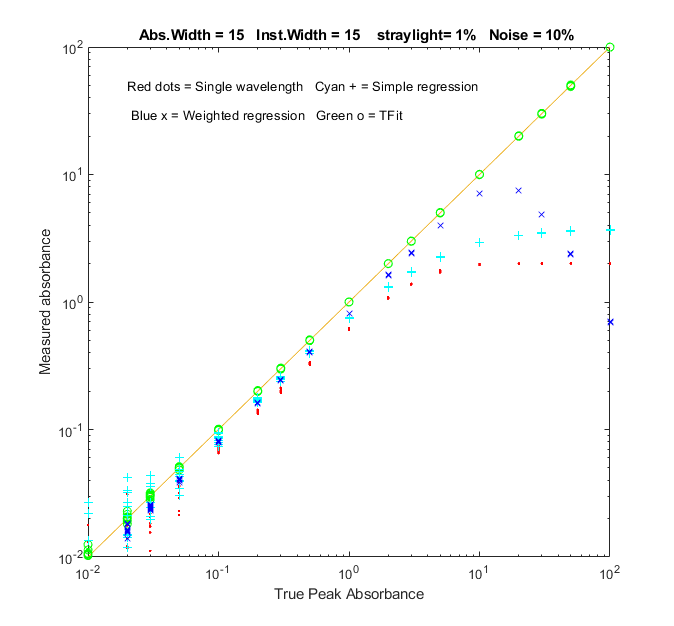Function that compares the analytical curves for single-wavelength, simple regression, weighted regression, and the TFit method over any specified absorbance range (specified by the vector "absorbancelist" in line 20). Simulates photon noise, unabsorbed stray light and random background intensity shifts. Plots a log-log scatter plot with each repeat measurement plotted as a separate point (so you can see the scatter of points at low absorbances). The parameters can be changed in lines 20 - 27.

In the sample result shown on the left, analytical curves for the four methods are computed over a 10,000-fold range, up to a peak absorbance of 100, demonstrating that the TFit method (shown by the green circles) is much more nearly linear over the whole range than the single-wavelength, simple regression, or weighted regression methods.The wide linearity range of Tfit is especially important in regulated laboratories where anything but linear least-squares fits to the calibration curve are discouraged.

This calibration curve function is included as a keypress command (M key) in TFitDemo.m.

Application to a three-component mixture (TFit3Demo.m or TFitDemoOctave.m)

The application of absorption spectroscopy to mixtures of absorbing components requires the adoption of one additional assumption: that of additivity of absorbances, meaning that the measured absorbance of a mixture be equal to the sum of the absorbances of the separate components.  In practice, this requires that the absorbers do not interact chemically; that is, that the do not react with themselves or with the other components or modify any property of the solution (e.g. pH, ionic strength, density, etc) that might effect the spectra of the other components. These requirements apply equally to the conventional multicomponent methods as well as to the T-Fit method.

TFit3.m is a demonstration of the T-Fit method applied to the multi-component absorption spectroscopy of a mixture of three absorbers. The adjustable parameters are: the absorbances of the three components (A1, A2, and A3), spectral overlap between the component spectra ("Sepn"), width of the instrument function ("InstWidth"), and the noise level ("Noise"). Compares quantitative measurement by weighted regression and TFit methods. Simulates photon noise, unabsorbed stray light and random background intensity shifts. Note: After executing this m-file, slide the "Figure No. 1" and "Figure No.2" windows side-by-side so that they don't overlap. Figure 1 shows a log-log scatter plot of the true vs. measured absorbances, with the three absorbers plotted in different colors and symbols. Figure 2 shows the transmission spectra of the three absorbers plotted in the corresponding colors. As you use the keyboard commands (below) in Figure No. 1, both graphs change accordingly.

In the sample calculation shown above, component 2 (shown in blue) is almost completely buried by the stronger absorption bands of components 1 and 3 on either side, giving a much weaker absorbance (0.1) than the other two components (3 and 5, respectively). Even in this case, the TFit method gives a result (T2=0.101) within 1% of the correct value (A2=0.1).  In fact, over most combinations of the three concentrations, the TFit methods works better (although of course nothing works if the spectral differences between the components is too small). Note: in this program, as in all of the above, when you change InstWidth, the photon noise is automatically changed accordingly just as it would in a real variable-slit dispersive spectrophotometer.

TFit3Demo.m and TFit3DemoOctave use keystrokes to interactively control the simulation parameters. Both versions use the same keystrokes.

KEYBOARD COMMANDS
A1           A/Z   Increase/decrease true absorbance of component 1
A2           S/X   Increase/decrease true absorbance of component 2
A3           D/C   Increase/decrease true absorbance of component 3
Sepn         F/V   Increase/decrease spectral separation of the
components

InstWidth    G/B   Increase/decrease width of instrument function
(spectral bandpass)

Noise        H/N   Increase/decrease random noise level when
InstWidth = 1

Peak shape   Q     Toggles between Gaussian and Lorentzian
absorption peak shape

Table        Tab   Print table of results
K     Print this list of keyboard commands

Sample table of results (by pressing the Tab key):
---------------------------------------------------------------
True        Weighted        TFit
Absorbance    Regression      method
Component 1      3             2.06          3.001
Component 2      0.1           0.4316        0.09829
Component 3      5             2.464         4.998Created October 03, 2006.  Revised December, 2021.
(c) Tom O'Haver
Professor Emeritus
Department of Chemistry and Biochemistry
The University of Maryland at College Park
toh@umd.edu
http://terpconnect.umd.edu/~toh/This page is part of "A Pragmatic Introduction to Signal Processing", created and maintained by Prof. Tom O'Haver , Department of Chemistry and Biochemistry, The University of Maryland at College Park. Comments, suggestions and questions should be directed to Prof. O'Haver at toh@umd.edu. Updated September 2020.